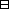## Group Theory and Linear Algebra

Last updated: 21 September 2014

## Lecture 16: Orthogonal complements and adjoints

Let $V$ be a vector space over $ℂ\text{.}$ Let $⟨,⟩:V×V\to ℂ$ be a positive definite Hermitian form. Let $W$ be a subspace of $V\text{.}$ The orthogonal complement to $W$ is $W⊥= { v∈V | if w∈W then ⟨v,w⟩ =0 } .$

 (a) ${W}^{\perp }$ is a subspace of $V\text{.}$ (b) $V=W\oplus {W}^{\perp }\text{.}$Proof.

(a)
To show:
 (aa) If ${u}_{1},{u}^{2}\in {W}^{\perp }$ then ${u}_{1}+{u}^{2}\in {W}^{\perp }\text{.}$ (ab) If $u\in {W}^{\perp }$ and $c\in ℂ$ then $cu\in {W}^{\perp }\text{.}$
 (aa) Assume ${u}_{1},{u}^{2}\in {W}^{\perp }\text{.}$ To show: ${u}_{1}+{u}^{2}\in {W}^{\perp }\text{.}$ To show: If $w\in W$ then $⟨{u}_{1}+{u}^{2},w⟩=0\text{.}$ Assume $w\in W\text{.}$ To show: $⟨{u}_{1}+{u}^{2},w⟩=0\text{.}$ $⟨u1+u2,w⟩ = ⟨u1,w⟩+ ⟨u2,w⟩ = 0+0,since u1,u2 ∈W⊥ = 0.$ (ab) Assume $u\in {W}^{\perp }$ and $c\in ℂ\text{.}$ To show: $cu\in {W}^{\perp }\text{.}$ To show: If $w\in W$ then $⟨cu,w⟩=0\text{.}$ Assume $w\in W\text{.}$ To show: $⟨cu,w⟩=0\text{.}$ $⟨cu,w⟩ = c⟨u,w⟩ = c·0,since u∈W⊥ = 0.$
(b)
To show: $V=W\oplus {W}^{\perp }\text{.}$
To show:
 (ba) $W\cap {W}^{\perp }=\left\{0\right\}\text{.}$ (bb) $W+{W}^{\perp }=V\text{.}$
Choose an orthonormal basis of $W$ (by Gram-Schmidt).
Extend this to an orthonormal basis of all $V$ (by more Gram-Schmidt). ${ b1,b2,…,bk ⏞basis of W , bk+1, bk+2,…, bk+ℓ ⏟basis of V }$ Then $\left\{{b}_{k+1},{b}_{k+2},\dots ,{b}_{k+\ell }\right\}$ is an orthonormal basis of ${W}^{\perp }\text{:}$ If $w={c}_{1}{b}_{1}+\cdots +{c}_{k}{b}_{k}\in W$ then $⟨bk+i,w⟩ = ⟨ bk+i, c1b1+⋯+ckbk ⟩ = c1⟨bk+i,b1⟩+⋯+ ck⟨bk+i,bk⟩ = c1·0+⋯+ck·0 = 0,$ so that ${b}_{k+i}\in {W}^{\perp }\text{.}$

$\square$

Let $V$ be a vector space over $C$ and $⟨,⟩:V×V\to ℂ$ a positive definite Hermitian form. Let $f:V\to V$ be a linear transformation. The adjoint of $f$ is a linear transformation ${f}^{*}:V\to V$ such that if $u,w\in V$ then $⟨f\left(u\right),w⟩=⟨u,{f}^{*}\left(w\right)⟩\text{.}$

The linear transformation $f:V\to V$ is

 $•$ self adjoint, or Hermitian, if $f$ satisfies $f=f*,$ $•$ an isometry, or unitary, if $f$ satisfies $f*f=1,$ $•$ normal, if $f$ satisfies $f*f=ff*.$

Let $V$ be a finite dimensional vector space over $ℂ$ and $⟨,⟩:V×V\to ℂ$ a positive definite Hermitian form. Let $f:V\to V$ be a linear transformation and $B=\left\{{b}_{1},\dots ,{\beta }_{k}\right\}$ an orthonormal basis of $V\text{.}$ Then $Bf*=(Bf‾)t.$

If $A$ is a matrix with $\left(i,j\right)$ entry ${A}_{ij}$ then ${A}^{t}$ is a matrix with $\left(i,j\right)$ entry ${A}_{ji}\text{.}$

Let $A$ be a matrix. The transpose of $A$ is the matrix ${A}^{t}$ given by $(At)ij=Aji.$ The conjugate of $A$ is the matrix $\stackrel{‾}{A}$ given by $(A‾)ij =Aij‾.$ The conjugate transpose of $A$ is the matrix ${\stackrel{‾}{A}}^{t}$ given by $(A‾t)ij =Aji‾.$Proof of the theorem. If $f*(bj)= p1jb1+ p2jb2+⋯+ pkjbk$ then $pij = ⟨f*(bj),bi⟩ = ⟨bi,f*(bj)⟩‾ = ⟨f(bi),bj⟩‾ = ⟨qi1+b1+q2ib2+⋯+qkibk,bj⟩‾ = qji‾.$ So ${B}_{{f}^{*}}=\left({p}_{ij}\right)$ and ${B}_{f}=\left({q}_{ij}\right)$ and $(Bf*)ij= pij=qji‾ =(Bf‾t)ij.$ So ${B}_{{f}^{*}}={\stackrel{‾}{{B}_{f}}}^{t}\text{.}$ $\square$

Let $V$ be a vector space over $ℂ$ which is finite dimensional and let $⟨,⟩:V×V\to ℂ$ be a positive definite Hermitian form. Let $f:V\to V$ be a linear transformation. Let $g:V\to V$ be a linear transformation. Then

 (a) ${f}^{*}V\to V$ is a linear transformation and is unique, (b) ${f}^{*}+{g}^{*}={\left(f+g\right)}^{*},$ (c) ${\left(fg\right)}^{*}={g}^{*}{f}^{*}\text{,}$ (d) If $c\in ℂ$ then ${\left(cf\right)}^{*}=\stackrel{‾}{c}{f}^{*},$ (e) ${\left({f}^{*}\right)}^{*}\text{.}$Idea of proof.

 (a) ${f}^{*}$ has matrix ${B}_{{f}^{*}}={\left(\stackrel{‾}{{B}_{f}}\right)}^{t}$ as given in the theorem.
Let $B=\left\{{b}_{1},\dots ,{b}_{k}\right\}$ be an orthonormal basis and let $A=Bf*and C=Bg*.$ Then show that
 (b') ${\left(\stackrel{‾}{A+C}\right)}^{t}={\stackrel{‾}{A}}^{t}+{\stackrel{‾}{C}}^{t},$ (c') ${\left(\stackrel{‾}{AC}\right)}^{t}={\stackrel{‾}{C}}^{t}{\stackrel{‾}{A}}^{t},$ (d') If $c\in C$ then ${\left(\stackrel{‾}{cA}\right)}^{t}=\stackrel{‾}{c}{\stackrel{‾}{A}}^{t},$ (e') ${\stackrel{‾}{\left({\stackrel{‾}{A}}^{t}\right)}}^{t}=A\text{.}$

$\square$

## Notes and References

These are a typed copy of Lecture 16 from a series of handwritten lecture notes for the class Group Theory and Linear Algebra given on August 30, 2011.